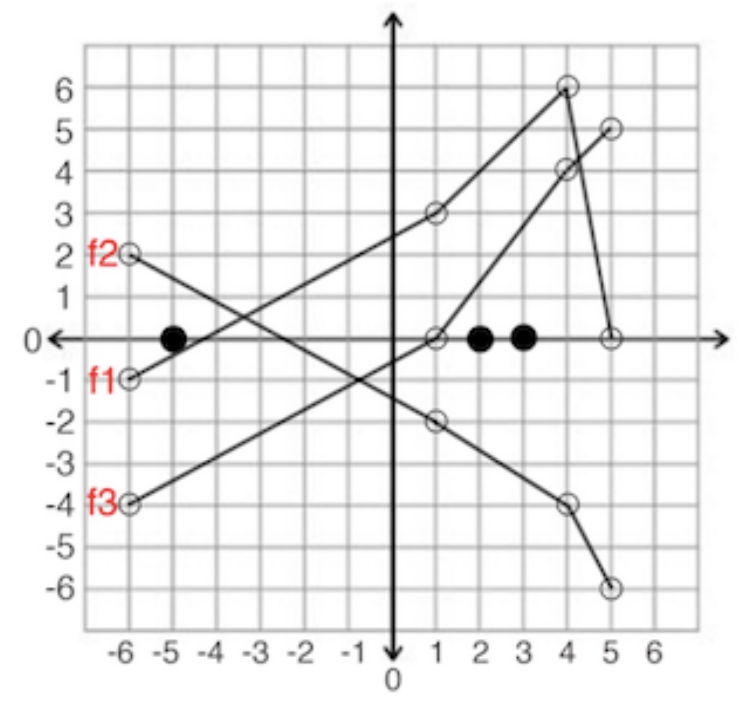시간 제한 메모리 제한 제출 정답 맞은 사람 정답 비율
2 초 512 MB 5 1 1 20.000%

## 문제

An army is going into war, and they want to divide all soldiers into some groups, in a way that maximizes their total strength. Let’s consider the N soldiers as points in a 2-dimensional plane, with all soldiers standing on the x-axis at distinct locations, the i-th soldier will be standing at xi on the x-axis (the soldiers are numbered from 1 to N). You will be given the soldiers in a sorted order based on their x value, from left to right.

Your task is to divide them into one or more groups, where each soldier belongs to exactly one group, and all members of any group are next to each other without anyone else from other groups in between them, so each group will be defined using 2 integers, a and b (where a ≤ b), which means this group includes all soldiers from the a-th position to the b-th position (inclusive).

Each soldier will be given a function fi to be used (only if that soldier is the left most soldier of a group) to evaluate the strength of the group. You will be given a list of M different values, each value is zj (they are numbered from 1 to M), which will be used to evaluate all the functions, for each function fi you will be given the value of fi(zj). To get the value of any z other than the given M ones, you just consider (zj, fi(zj)) as a point, and connect every 2 consecutive points (based on zj) in each function using a straight line segment, and now you have a function which covers all possible values from z1 to zM.

The strength of the whole army is the sum of strengths of each group, the strength of a group from the a-th position to the b-th position is fa(xb), in other words, it’s the value of the function for the left most soldier when we pass the x value of the right most soldier to it. Check the notes at the end for more explanation of the first test case.

You are given all the required details as described above, and your task is to divide the soldiers into groups to maximize the total strength of the whole army.

## 입력

Your program will be tested on one or more test cases. The first line of the input will be a single integer T (1 ≤ T ≤ 100) representing the number of test cases. Followed by T test cases.

Each test case starts with a line containing 2 integers separated by a space, N (1 ≤ N ≤ 105) representing the number of soldiers and M (2 ≤ M, N × M ≤ 105) representing the number of z values.

Followed by a line containing N sorted integers separated by a space, which are the positions of the soldiers x1, x2, . . . , xN (-106 ≤ xi ≤ 106).

Followed by a line containing M sorted integers separated by a space, which are the z values as described above z1, z2, . . . , zM (-106 ≤ zj ≤ 106).

Followed by N lines, each line contains M integers separated by a space. The j-th value from the left in the i-th line from the top is the value of fi(zj) (-106 ≤ fi(zj) ≤ 106).

It’s guaranteed that z1 ≤ x1 and xN ≤ zM.

## 출력

For each test case print a single line containing a single decimal number rounded to exactly 6 decimal places, which is the maximum strength of the whole army you can get.

## 예제 입력 1

3
3 4
-5 2 3
-6 1 4 5
-1 3 6 0
2 -2 -4 -6
-4 0 4 5
5 2
-2 5 8 9 10
-2 10
-7 -6
-3 -7
0 -8
9 -10
5 -4
2 2
0 7
-2 7
-10 -2
-4 -10


## 예제 출력 1

6.666667
-6.000000
-2.000000


## 힌트

The following image represents the first test case:The 3 solid circlers on the x-axis are the locations of the 3 soldiers, and we have the 3 functions f1, f2 and f3 (one for each soldier) plotted as described above. The best solution here is to put the first 2 soldiers in a group, the strength of that group will be f1(x2), which is f1(2) = 4, and the last soldier alone and the strength of that group will be f3(x3),which is f3(3) = 2 .666667, so the total strength is 6.666667 (everything rounded to 6 decimal places).